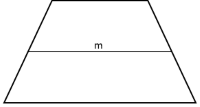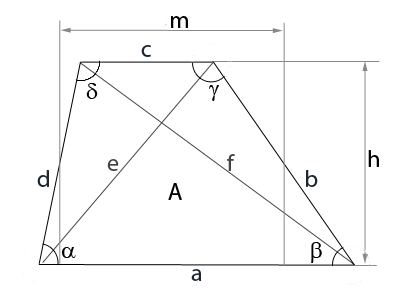# Trapezoid calculation

Description and formulas for the calculation of trapezoids

## Trapezoid definition

A trapezoid is a quadrangular geometric shape with the following characteristics

• A trapezoids have one pair of parallel sides, which are the bases of the trapezoid

• The opposite sides are different in length• The midsegment $$m$$ of a trapezoid is parallel to each base. Its measure is one half of the sum of the measures of the bases

• The Figure above shows an isosceles trapezoid. The sides are also congruent. As seen in the figure, an isosceles trapezoid has two pairs of congruent angles, the diagonals are congruent, and it has one line of symmetry

## Legend

$$a$$ Side length a

$$b$$ Side length b

$$c$$ Side length c

$$d$$ Side length d

$$e$$ Diagonal e

$$f$$ Diagonal f

$$h$$ Height

$$m$$ Midsegment

$$A$$ Area

$$P$$ Perimeter

$$α$$ Angle Alpha

$$β$$ Angle Beta

$$γ$$ Angle Gamma

$$δ$$ Angle Delta## Formulas for Trapezoid calculation

#### Calculate side length $$a$$ of a Trapezoid$$\displaystyle a = (A · 2) / h -c$$

$$a = m · 2 -c$$

#### Calculate side length $$b$$ of a Trapezoid$$\displaystyle b =h / sin(β)$$

$$b = h / sin(γ)$$

#### Calculate side length $$c$$ of a Trapezoid$$\displaystyle c = (A · 2 / h) - a$$

$$c = m · 2 - a$$

#### Calculate side length $$d$$ of a Trapezoid$$\displaystyle d = h / sin(α)$$

$$d = h / sin(δ)$$

#### Calculate diagonal $$e$$ of a Trapezoid$$\displaystyle e = \sqrt{a^2 + b^2 - 2 · a · b · cos(β)}$$

#### Calculate diagonal $$f$$ of a Trapezoid$$\displaystyle f = \sqrt{a^2 + d^2 - 2 · a · d · cos(α)}$$

#### Calculate Height $$h$$ of a Trapezoid$$\displaystyle h = (2 · a) / (a + c)$$

$$h = b · sin(β)$$

#### Calculate Midsegment $$m$$ of a Trapezoid$$\displaystyle m = (a + c) / 2$$

$$m = A / h$$

#### Calculate Area $$A$$ of a Trapezoid$$\displaystyle A = (a + c) / 2 · h$$

$$A = m · h$$

#### Calculate Perimeter $$P$$ of a Trapezoid$$\displaystyle P = a + b + c+ d$$

#### Calculate Angle Alpha $$α$$ of a Trapezoid$$\displaystyle α = asin(h / d)$$

$$\displaystyle α = 180 - δ$$

#### Calculate Angle Beta $$β$$ of a Trapezoid$$\displaystyle β = asin(h / b)$$

$$\displaystyle β = 180 - γ$$

#### Calculate Angle Gamma $$γ$$ of a Trapezoid$$\displaystyle γ = 180 - β$$

#### Calculate Angle Delta $$δ$$ of a Trapezoid$$\displaystyle δ = 180 - α$$